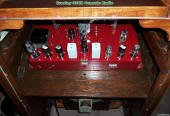# Amplitude ModulationAmplitude modulation uses the instantaneous amplitude of a modulating signal (voice, music, data, etc.) to directly vary the amplitude of a carrier signal. Modulation index, m, is used to describe the ratio of maximum voltage to minimum voltage in the modulated signal. If the modulating signal is equal in magnitude to the carrier, then m = 1 and the modulated signal varies from a scaled maximum of unity down to zero (see figure below). When m = 0, no modulation of the carrier is performed. If m is greater than 1, the carrier is actually cut off for some period of time, and unwanted harmonics are created at the transmitter output.

The pictures here are of my restored Crosley 03CB radio, which was manufactured in 1941, before commercial FM broadcast radio was still in the experimental phase of development. This radio tuned the 540 to 1700 kHz AM band and the 16 to 49 meter shortwave band.

 Class ConductionAngle MaximumEfficiency Description A 360º 25% Device is biased in to conduct in the linear region all the time. AB > 180º,<< 360º --- Two devices connected like a Class B, but biased to conduct somewhere between Class A and Class B. B 180º 78.5% Two devices in series with the output taken at the common junction. Both devices biased to conduct in the linear region for opposite half a cycle, i.e., they are never on at the same time. C > 0º,< 180º --- Device is biased to turn on after a certain input threshold voltage is exceeded. Very efficient, but creates distortion. D 100% Used to switch completely on or completely off. E Used for rectangular input signals.

In the frequency domain, the carrier frequency is flanked on both sides by mirror image copies of the modulating signal.

M1 = Ωc ± ωm1, ΩM2 = Ωc ± Ωm2AM General Equation

Let the carrier be xc(t) = Xc·sin (Ωct), and the modulating signal be xm(t) = Xm·sin (ωmt)

Then   x(t) = Xc·[1+m·sin (Ωmt)]·sin (Ωct)

Modulation IndexIn the following examples, the carrier frequency is nine times the modulation frequency. Red (dashed) lines represent the modulation envelope. Blue (solid) lines represent the modulated carrier.

 100% ModulationHere, the maximum voltage (Vmax) is 2 V and the minimum (Vmin) is 0 V. From the modulation index formula:50% ModulationHere, the maximum voltage (Vmax) is 3 V and the minimum (Vmin) is 1 V. From the modulation index formula:25% ModulationHere, the maximum voltage (Vmax) is 1.25 V and the minimum (Vmin) is 0.75 V. From the modulation index formula:150% ModulationHere, the maximum voltage (Vmax) is 2.5 V and the minimum (Vmin) is -0.5 V. From the modulation index formula:Note: AM waveforms created with MathCAD 4.0 software.About RF CafeCopyright: 1996 - 2024Webmaster:    Kirt Blattenberger,    BSEE - KB3UON RF Cafe began life in 1996 as "RF Tools" in an AOL screen name web space totaling 2 MB. Its primary purpose was to provide me with ready access to commonly needed formulas and reference material while performing my work as an RF system and circuit design engineer. The Internet was still largely an unknown entity at the time and not much was available in the form of WYSIWYG ... All trademarks, copyrights, patents, and other rights of ownership to images and text used on the RF Cafe website are hereby acknowledged. My Hobby Website:  AirplanesAndRockets.com